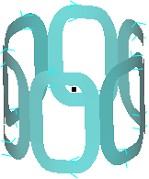# Coil for rotating machine applications

## Shape and dimension

The complex coils proposed to the user for rotating machine applications are the following ones:Dimensions of a saddle coil are presented on an example in the following block.

## Example

An example of a saddle coil is presented above.A saddle coil is defined by following parameters:

• Opening angle of the straight part: φ = 25°

• Opening angle of the cylindrical part: ψ = 20°

• Length of the straight part of the saddle: L = 30

• Semi internal height of the saddle: H = 30